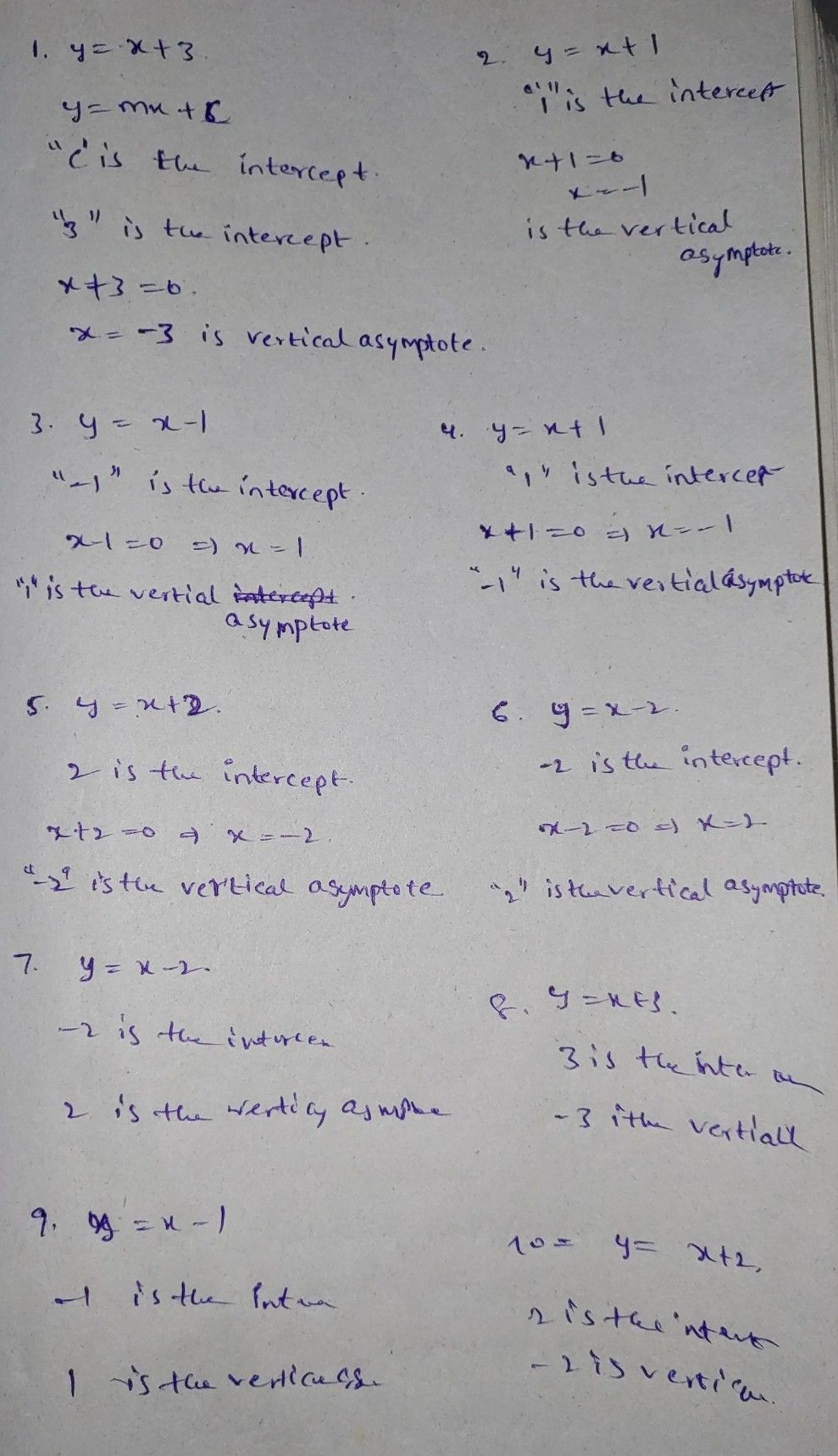Symbol
ProblemAdditional Activities Determine the intercept, zero and vertical asymptote of the following logarithmic functions. Write your answer in a sheet of paper. $1.y=\left(x+3\right)$ $6.y=x-2$ $2.y=x+1$ $7$ $y=\left(x-2\right)$ $3.y=\left(x-1\right)$ $8.y=x+3$ $4.y=\left(x+1\right)$ $9.y=x-1$ $5.y=x+2$ $10.y=\left(x+2\right)$
10th-13th grade
Other
Search count: 110
SolutionQanda teacher - Sairaj439Student
thank you so much godbless you